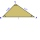# Pythagorean theorem + expression of a variable from formula - problems

1. Three altitudesA triangle with altitudes 4; 5 and 6 cm is given. Calculate the lengths of all medians and all sides in a triangle.
2. Right ΔA right triangle has the length of one leg 28 cm and length of the hypotenuse 53 cm. Calculate the height of the triangle.
3. Cube in a sphereThe cube is inscribed in a sphere with volume 9921 cm3. Determine the length of the edges of a cube.
4. Cone A2VSurface of cone in the plane is a circular arc with central angle of 126° and area 415 cm2. Calculate the volume of a cone.
5. MO SK/CZ Z9–I–3John had the ball that rolled into the pool and it swam in the water. Its highest point was 2 cm above the surface. Diameter of circle that marked the water level on the surface of the ball was 8 cm. Determine the diameter of John ball.
6. Square diagonalCalculate length of the square diagonal if the perimeter is 476 cm.
7. IS trapezoidCalculate the length of diagonal u and height v of isosceles trapezoid ABCD, whose bases have lengths a = |AB| = 37 cm, c = |CD| = 29 cm and legs b = d = |BC| = |AD| = 28 cm.
8. Right triangleLegs of right are in ratio a:b = 2:8. Hypotenuse has a length of 87 cm. Calculate the perimeter and area of the triangle.
9. Floating barrelBarrel (cylinder shape) floats on water, top of barrel is 8 dm above water and the width of surfaced barrel part is 23 dm. Barrel length is 24 dm. Calculate the volume of the barrel.
10. R TrapeziumRectangular trapezium has bases 12 and 5 and area 84 cm2. What is its perimeter?
11. ISO triangleCalculate the area of an isosceles triangle KLM if the length of its sides are in the ratio k:l:m = 4:4:3 and has perimeter 377 mm.
12. Rhombus 2Calculate the area of rhombus which has a height v=48 mm and shorter diagonal u = 60 mm long.
13. Right isoscelesCalculate area of the isosceles right triangle which perimeter is 41 cm.
14. Square diagonalCalculate the length of diagonal of the square with side a = 23 cm.
15. Right triangleCalculate the missing side b and interior angles, perimeter and area of ​​a right triangle if a=10 cm and hypotenuse c = 16 cm.
16. Perimeter and legsDetermine the perimeter of a right triangle if the length of one leg is 75% length of the second leg and its content area is 24 cm2.
17. Cone and the ratioRotational cone has a height 23 cm and the ratio of the base surface to lateral surface is 7: 9. Calculate a surface of the base and the lateral surface.
18. Trapezoidtrapezoid ABCD a = 35 m, b=28 m c = 11 m and d = 14 m. How to calculate its area?
19. Rectangle diagonalsIt is given rectangle with area 24 cm2 a circumference 20 cm. The length of one side is 2 cm larger than length of second side. Calculate the length of the diagonal. Length and width are yet expressed in natural numbers.
20. Nice prismCalculate the surface of the cuboid if the sum of its edges is a + b + c = 19 cm and the body diagonal size u = 13 cm.

Do you have an interesting mathematical problem that you can't solve it? Enter it, and we can try to solve it.

To this e-mail address, we will reply solution; solved examples are also published here. Please enter e-mail correctly and check whether you don't have a full mailbox.

Pythagorean theorem is the base for the right triangle calculator.